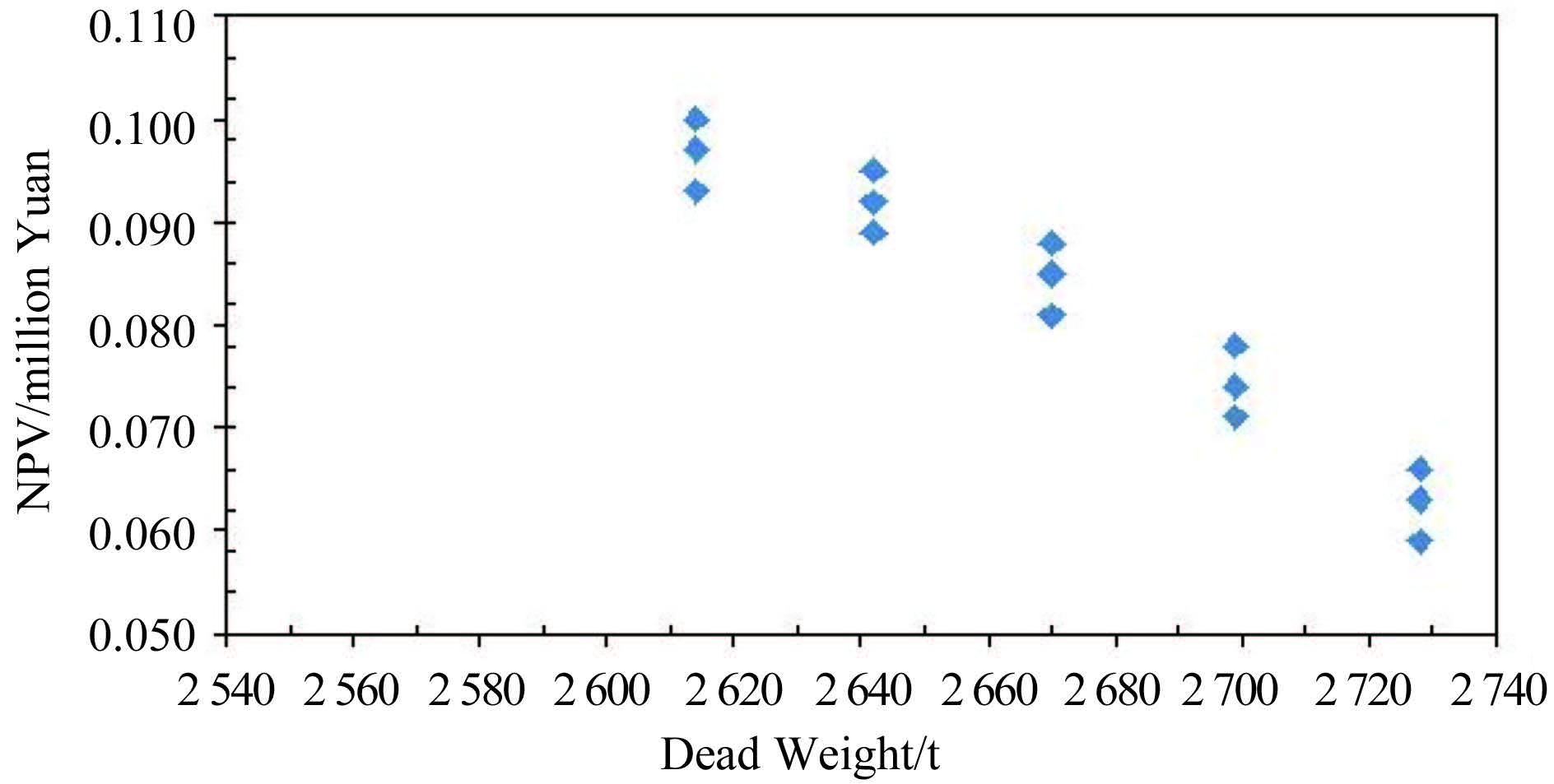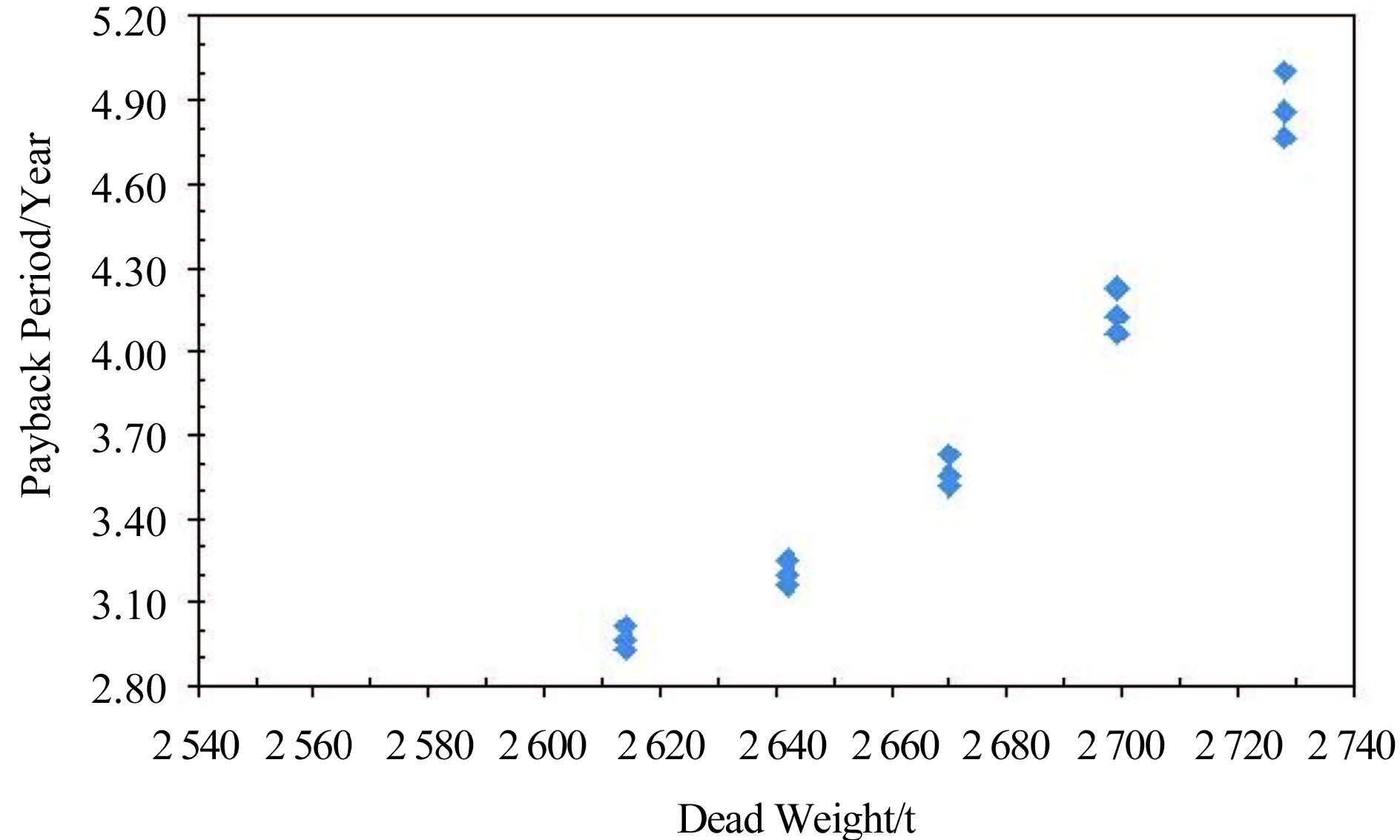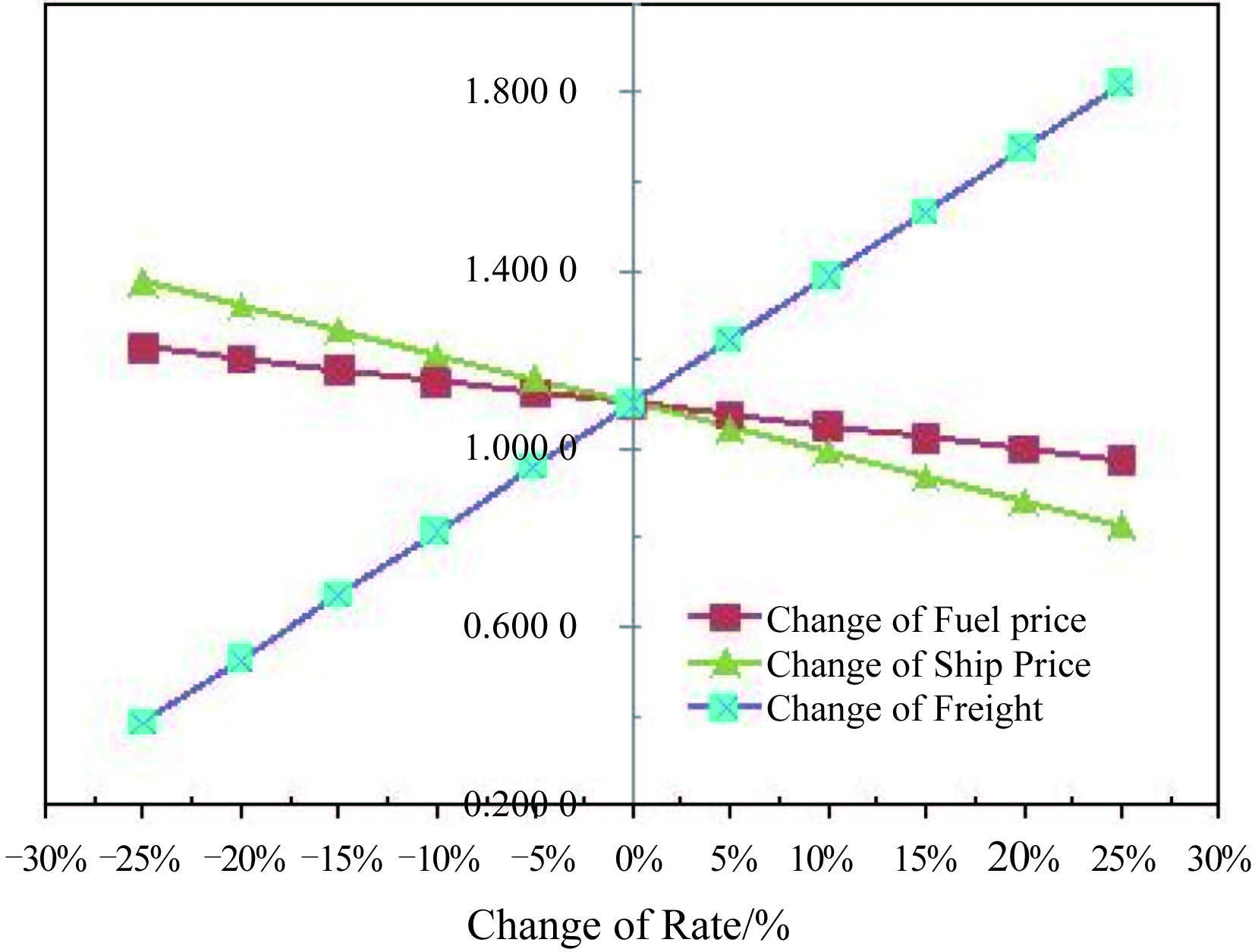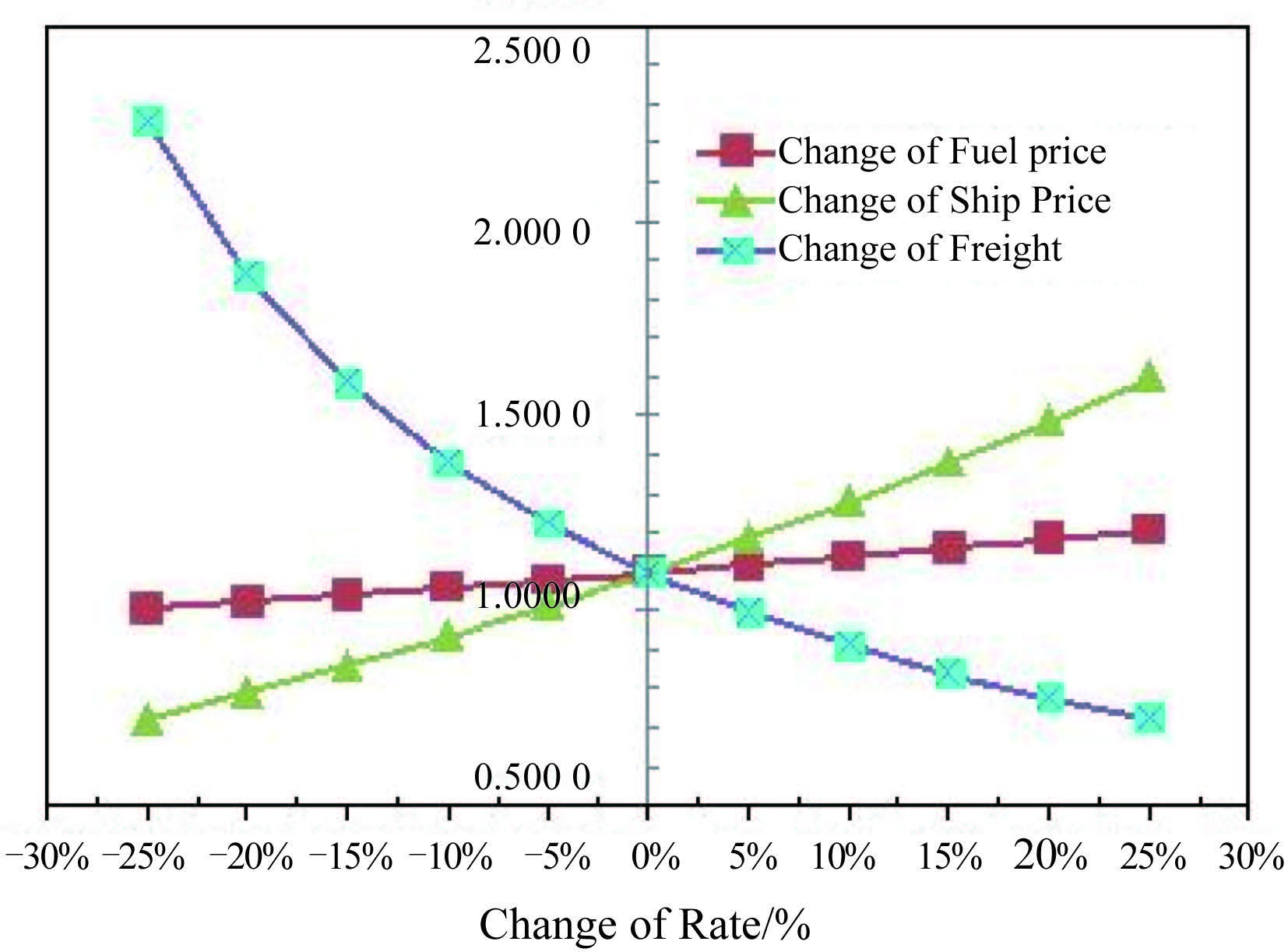﻿ 基于变值法的船型优化层次分析法模型研究
 舰船科学技术2016, Vol. 38Issue (5): 69-73,78PDF

1. 广州航海学院 船舶工程学院, 广东 广州 510725 ;
2. 上海交通大学 船舶海洋与建筑工程学院, 上海 200240 ;
3. 广东工业大学 自动化学院, 广东 广州 510006

Research on the analytic hierarchy process model of ship optimization based on variable value method
CHEN Jian-ping1,2, XU Jie3, GONG You1, HUANG Peng-ju1, XU Yan-min1
1. School of Ship Engineering, Guangzhou Maritime Institute, Guangzhou 510725, China ;
2. School of Naval Architecture, Ocean and Civil Engineering, Shanghai Jiaotong University, Shanghai China 200240, China ;
3. Faculty of Automation, Guangdong University of Technology, Guangzhou 510006, China
Abstract: The paper presents the Analytic Hierarchy Process method of ship hull form technology and economy, and establishes a mathematical model for the economic evaluation of ship hull form by employing the theory of Analytic Hierarchy Process and variable value method. Based on the case study of the ship type of the 3 000 ton bulk cargo ship in the Pearl River main line, the paper obtains the sort of optimization comprehensive evaluation index, and obtains the comprehensive optimal ship form. On this basis, it studies the sensitivity analysis of the parameters effecting on the ship form scheme. The economic evaluation method of Analytic Hierarchy Process proposed has theoretical and practical value for the design and optimization of ship form optimization.
Key words: ship form optimization     analytic hierarchy process     variable value method     sensitivity analysis
0 前言

1 船舶技术和经济性评价

1）初始数据处理。对船舶的类型、营运、造价等方面进行收集调查，对原始数据进行分析，得出船舶技术经济参数的评价过程

2）船舶多方案技术和经济指标计算。根据船舶运营实际情况，确定典型的航线和典型的船舶吨位、船型方案的性能计算。

3）船舶类型评价。首先确定船舶技术综合评价指标，然后根据评价指标，采用多目标综合评价法对船舶技术经济综合评价指标进行综合评价。

4）确定最佳船型。根据船舶综合排序的结果，确定船舶类型和典型船型。

5）敏感性分析。对典型船型的技术经济指标进行敏感性分析。

2 船型优化模型 2.1 层次分析法

2.2 建立模型 2.2.1 构建模型层次

2.2.2 构建判断矩阵

 \left\{ \begin{align} & {{a}_{ij}}>0, \\ & {{a}_{ij}}={}^{1}\!\!\diagup\!\!{}_{{{a}_{ji}}}\;, \\ & {{a}_{ii}}=1. \\ \end{align} \right. (1)
2.2.3 层次单排序及其一致性

 ${{AV}} = {\lambda _{\max }}{{V}}$ (2)

 $\begin{array}{*{35}{l}} {{a}_{ij}}=\frac{{{a}_{ik}}}{{{a}_{jk}}},\left( i=1,2,\cdots ,m;j=1,2,\cdots ,m;k=1,2,\cdots ,m \right). \\ \end{array}$ (3)表 1 1～9 阶判断矩阵 RI Tab.1 Mean random consistency index of judgment matrix
2.2.4 层次总排序和一致性检验表 2 总排序计算表 Tab.2 Total sorting table

 $CR = \frac{{\sum\limits_{j = 1}^m {{a_j}C{I_j}} }}{{\sum\limits_{j = 1}^m {{a_j}R{I_j}} }}.$ (4)

CR＜0.10，即认为总排序结果满足一致性。

3 算例表 3 船舶基本参数 Tab.3 Ship basic parameters
3.1 船型参数选取

 ${C_B} = \displaystyle\frac{\Delta }{{k \times \rho \times {L_{pp}} \times B \times T}},$

DW=IDWRCW×CW

 $V=\sqrt{\frac{{{C}_{E}}\times {{P}_{E}}}{{{\Delta }^{2/3}}}},$

 $P=0.0884{{\text{e}}^{0.0102{{L}_{pp}}}}{{L}_{pp}}\cdot B\cdot D.$

 $ATC=RIY\times \left( D{{W}_{down-flow}}+D{{W}_{contra-flow}} \right),$

 $PBP=\log \left( A/\left( A-P\times i \right) \right)/\log \left( 1+i \right),$

 $NPV = \left( {B - Y} \right)\left( {P/A,i\% ,n} \right) + \Delta P\left( {P/F,i\% ,n} \right) - P.$

3.2 变值法（网格法）构造船型方案表 4 变值法构造船型方案 1 Tab.4 Ship form scheme with variable value method 1表 5 变值法构造船型方案 2 Tab.5 Ship form scheme with variable value method 2
3.3 计算结果表 6 船型评价指标计算结果 1 Tab.6 The evaluation index ranking 1表 7 船型评价指标计算结果 2 Tab.7 The evaluation index ranking 2图 1 净现值（NPV）分布 Fig. 1 The distribution of NPV图 2 投资回收期（PBP）分布 Fig. 2 The distribution of PBP
4 敏感性分析图 3 投资回收期（PBP）的敏感性变化曲线 Fig. 3 The sensibility analysis curve of PBP图 4 净现值（NPV）的敏感性变化曲线 Fig. 4 The sensibility analysis curve of NPV
5 结语

  杨路春, 李学斌, 丁明君, 等. 多目标遗传算法和决策在船型论证中的应用[J]. 哈尔滨工程大学学报 , 2012, 32 (12) :1459–1464. YANG Lu-chun, LI Xue-bin, DING Ming-jun, et al. Application of multi-objective genetic algorithm and decision-making skills in ship form evaluation[J]. Journal of Harbin Engineering University , 2012, 32 (12) :1459–1464.  程红蓉, 刘晓东, 冯佰威. 多目标优化在船型设计中的应用研究[J]. 中国造船 , 2014, 55 (1) :76–82. CHENG Hong-rong, LIU Xiao-dong, FENG Bai-wei. Study on multidisciplinary optimization method for hull forms design[J]. Shipbuilding of China , 2014, 55 (1) :76–82.  陈雅菊. 基于多目标遗传算法和主成分分析的船型主尺度论证[J]. 船海工程 , 2015, 44 (2) :31–35. CHEN Ya-ju. Application of fast non-dominated sorted genetic algorithm and principle component analysis in ship main dimensions design[J]. Ship & Ocean Engineering , 2015, 44 (2) :31–35.  陈继红. 基于灰色关联的船型决策经济论证方法与应用[J]. 中国航海 , 2012, 35 (2) :102–105. CHEN Ji-hong. Method of economic evaluation for ship form development decision making based on gray relational theory and its application[J]. Navigation of China , 2012, 35 (2) :102–105.  丁丽萍, 张晓晗. 必要费率在经济船型论证和方案比选中的应用[J]. 港工技术 , 2014, 51 (4) :63–65. DING Li-ping, ZHANG Xiao-han. Application of required rate in analysis of economical ship style and comparison of options[J]. Port Engineering Technology , 2014, 51 (4) :63–65.  李文龙, 谭家华. 基于最小偏差法的集装箱船船型优化[J]. 中国造船 , 2002, 43 (4) :1–5. LI Wen-long, TAN Jia-hua. Optimization of container ship principal parameters based on minimum-deviation method[J]. Shipbuilding of China , 2002, 43 (4) :1–5.  GUIN M M, KATO H, YAMAGUCHI H, et al. Reduction of skin friction by microbubbles and its relation with near-wall bubble concentration in a channel[J]. Journal of Marine Science and Technology , 1996, 1 (5) :241–254. DOI:10.1007/BF02390723  BESNARD E, SCHMITZ A, HEFAZI H, et al. Constructive neural networks and their application to ship multidisciplinary design optimization[J]. Journal of Ship research , 2007, 51 (4) :297–312.  陈衍泰, 陈国宏, 李美娟. 综合评价方法分类及研究进展[J]. 管理科学学报 , 2004, 7 (2) :69–79. CHEN Yan-tai, CHEN Guo-hong, LI Mei-juan. Classification & research advancement of comprehensive evaluation methods[J]. Journal of Management Sciences in China , 2004, 7 (2) :69–79.  刘寅东, 余秀丽, 苏绍娟. 西江干线集装箱船船型论证[J]. 船海工程 , 2011, 40 (2) :9–13. LIU Yin-dong, YU Xiu-li, SU Shao-juan. Technical and economic evaluation for the container ships in Xijiang river[J]. Ship & Ocean Engineering , 2011, 40 (2) :9–13.  SATTY T L. Applications of analytical hierarchies[J]. Mathematics and Computers in Simulation , 1979, 21 (1) :1–20. DOI:10.1016/0378-4754(79)90101-0  SAATY T L. Modeling unstructured decision problems:a theory of analytical hierarchy[C]//Proceedings of the First International Conference on Mathematical Modeling. Rolla:University of Missoure Rolla, 1997, 1:59-77.  SATTY T L. The analytical hierarchy process[M]. New York: McGraw-Hill Company, 1980 .  桑松.船型方案论证与智能决策方法研究[D].大连:大连理工大学, 2002. SANG Song. Study on ship form evaluate alternatives and intelligent decision-making methods[D]. Dalian:Dalian University of Technology, 2002. http://cdmd.cnki.com.cn/article/cdmd-10141-2003110418.htm